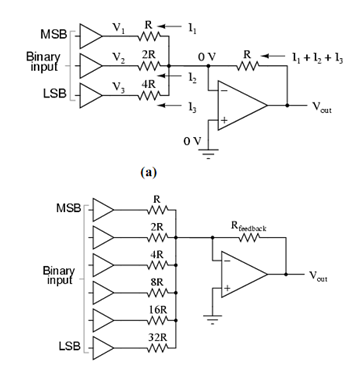## Digital to Analog Converter Assignment Help

Assignment Help: >> Digital to Analog and Analog to Digital Converters - Digital to Analog Converter

Digital to Analog Converter:

Suppose we were to set the input resistor values at multiple powers of two: R, 2R, and 4R of the comparator circuit of Figure (a). Beginning from V1 and going through V3, it would give each input voltage exactly half the effect on the output as the voltage before it. In other terms, input voltage V1 has a 1: 1 influenced on the output voltage (gain of 1), whereas input voltage V2 has half that much effect on the output (a gain of 1/2), & V3 half of that (a gain of 1/4). These ratios are not arbitrarily selected: they are the same ratios corresponding to place weights in the binary number system. If we drive the inputs of this circuit along with digital gates so that each of input is either 0 volts or full supply voltage, the output voltage will be an analog demonstration of the binary value of these three bits. If we desire to expand the resolution of this DAC (add more bits to the input), all we have to do is add more input resistors, holding up to the same power-of-two sequence of values as in Figure (b).(b)

Figure: (a) Simple 3-bit DAC; and (b) 6-bit Binary-weighted DAC